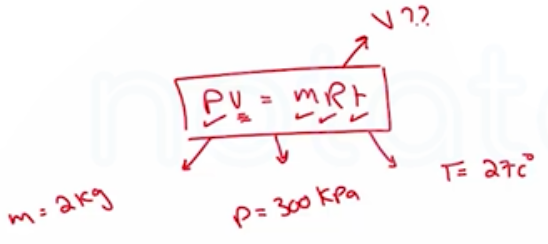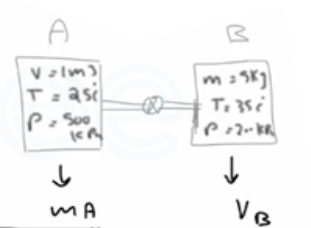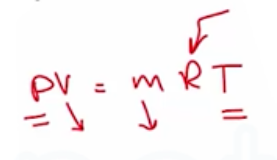Need Help?

Subscribe to Thermodynamics

###### \${selected_topic_name}
• Notes
• Comments & Questions

$\begin{array}{l}{\text { A } 2 \text { -kg mass of helium is maintained at } 300 \mathrm{kPa} \text { and } 27^{\circ} \mathrm{C} \text { in a rigid container. }} \\ {\text { How large is the container, in } \mathrm{m} 3 ?}\end{array}$

$\begin{array}{l}{3-76 \text { A spherical balloon with a diameter of } 9 \mathrm{m} \text { is filled }} \\ {\text { with helium at } 27^{\circ} \mathrm{C} \text { and } 200 \mathrm{kPa} \text { . Determine the mole number }} \\ {\text { and the mass of the helium in the balloon }}\end{array}$$\text { T. } A-1 \longrightarrow R=2.0769$

$T=27+273=300 \mathrm{k}$

$P v=m R T \longrightarrow V=\frac{m R T}{P}=\frac{2 * 2.0769*300}{300}$

$∴ {V}=4.154 \mathrm{m}^{3}$

$\begin{array}{l}{\text { A } 1-m 3 \text { tank containing air at } 25^{\circ} \mathrm{C} \text { and } 500 \mathrm{kPa} \text { is connected through }} \\ {\text { a valve to another tank containing } 5 \mathrm{kg} \text { of air at } 35^{\circ} \mathrm{C} \text { and } 200 \mathrm{kPa} \text { . }}\end{array}$

$\begin{array}{l}{\text { Now the valve is opened, and the entire system is allowed }} \\ {\text { to reach thermal equilibrium with the surroundings, which are at } 20^{\circ} \mathrm{C} \text { . }} \\ {\text { Determine the volume of the second tank and the final equilibrium pressure of air }}\end{array}$

$A-1 \longrightarrow R=0.287$$P v=m R T \rightarrow V_{B}=\frac{m R T_{1}}{P_{1}}$

$\longrightarrow \frac{5 * 0.287 * 308}{200}={2.21 m^{3}}$

$m_{A}=\frac{p v}{R T}=\frac{500*1}{0.287 * 298}={5.846}kg$

$\longrightarrow V=V_{A}+V_{B}=1+2 \cdot 21=3.21 \mathrm{m}^{3}$

$\longrightarrow M=m_{A}+m_{B}=5.846+5=10.846 \mathrm{kg}$

$P_{2}=\frac{m R T}{V}=\frac{10.846 * 0.287 * 293}{3.21}=284.1 kPa$

$\begin{array}{l}{\text { A rigid tank contains an ideal gas at } 1227^{\circ} \mathrm{C} \text { and } 200 \mathrm{kPa} \text { gage. }} \\ {\text { The gas is cooled until the gage pressure reads } 50 \mathrm{kPa} \text { . }}\end{array}$

$\text { If the atmospheric pressure is } 100 \mathrm{kPa} \text { , determine the final temperature of the gas }$$m_{1}=m_ 2$

$\frac{P_1 V_ 1}{T_{1}}=\frac{P_{2} V_{2}}{T_{2}} \rightarrow \frac{P_{1}}{T_1} = \frac{P_{2}}{T_{2}}$

$\longrightarrow \quad T_{2}=T_{1} \frac{P_{2}}{P_1}$

$=(1227+273) * \frac{(50+100)}{(200+100)}$

$=750 \mathrm{k}$

$=477 c^{\circ}$

No comments yet